Next: Mean, Variance, and Standard Up: Probability Theory Previous: Combinatorial Analysis

# Binomial Probability Distribution

It follows from Equations (2.16) and (2.20) that the probability of obtaining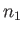occurrences of the outcome 1 in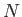statistically independent observations of a two-state system is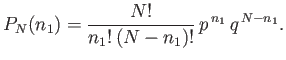(2.21)

This probability function is called the binomial probability distribution. The reason for this name becomes obvious if we tabulate the probabilities for the first few possible values of, as is done in Table 2.1. Of course, we immediately recognize the expressions appearing in the first four rows of this table: they appear in the standard algebraic expansions of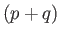,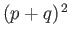,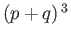, and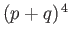, respectively. In algebra, the expansion of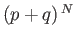is called the binomial expansion (hence, the name given to the probability distribution function), and is written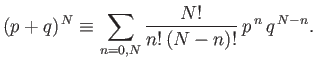(2.22)

Equations (2.21) and (2.22) can be used to establish the normalization condition for the binomial distribution function: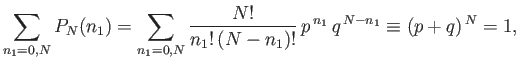(2.23)

because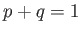. [See Equation (2.11).]

Table 2.1: The binomial probability distribution,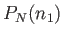.0 1 2 3 4 1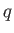2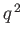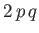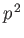3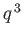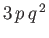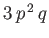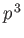4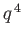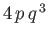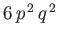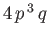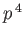Next: Mean, Variance, and Standard Up: Probability Theory Previous: Combinatorial Analysis
Richard Fitzpatrick 2016-01-25